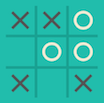## 273387 Swift - Mini Test 3The final class

Awaiting submission
You are given 2 ordered lists and must combine the elements into 1 ordered list. For example:
```let list1 = [2, 3, 7, 9]
let list2 = [1, 5, 6]
let combined = merge(list: list1, with: list2)   // [1, 2, 3, 5, 6, 7, 9]
```
Write the `merge` function that takes 2 parameters (both Int arrays) and returns the combined ordered list.

Use the following code as a template:

```// TODO: write your function here

print("Enter list 1:")
let list1 = (readLine() ?? "").components(separatedBy: ",").flatMap { Int(\$0)}
print("Enter list 2:")
let list2 = (readLine() ?? "").components(separatedBy: ",").flatMap { Int(\$0)}
let result: [Int] = merge(list: list1, with: list2)
print(result.map({ String(\$0) }).joined(separator: ","))
```
Awaiting submission
The amount of rain is calculated each month and stored in the `Rainfall` class. Complete the class such that it returns the rainfall for a month, the total rainfall over 12 months, the average rainfall over 12 months and the month with the highest rainfall. Use the following code as a template:
```class Rainfall {
var amounts: [Float]

init(amounts: [Float]) {
self.amounts = amounts
}

// TODO: write functions
}

print("Enter rainfall amounts:")
let list = (readLine() ?? "").components(separatedBy: ",").flatMap { Float(\$0)}
let rainfall = Rainfall(amounts: list)
print("July: \(rainfall.at(month: 6))")
print("Total: \(rainfall.total())")
print("Average: \(rainfall.average())")
print("Highest month: \(rainfall.highestMonth())")
```
Awaiting submission

Write a program that determines the winning move in a game of noughts and crosses (tic tac toe).In this example, it is O's turn and they can win by placing an O in column 1, row 2. The input to the program is a 3x3 grid and the current player. The output will be the column and row (1-3) of the winning move. If there is no winning move then return `NONE`.